• 1. 定义法由于原问题较简单，可以直接通过定义来求解特征值及特征向量。|A-xI|=0, 即化为简单的1元3次方程，求解的x=[-1 -2 -3].然后根据(A-xI)v=0,分别将以上三个值代入求解，记得3组特征向量。从而将a对角化。这个...

匿名用户

1级

2008-12-14 回答

我觉得这个问题可以有以下3种解法。

1. 定义法

由于原问题较简单，可以直接通过定义来求解特征值及特征向量。

|A-xI|=0, 即化为简单的1元3次方程，求解的x=[-1 -2 -3].

然后根据

(A-xI)v=0,分别将以上三个值代入求解，记得3组特征向量。从而将a对角化。

这个方法没有牵涉到特殊的矩阵运算，只有简单的解方程。matlab以及c实现都非常简单。

2. power method

这个方法比较适合小型问题的求解。以下是基于power method对该问题进行求解。可以直接求得特征值和特征向量。没有非常复杂的矩阵操作，可以用简单的matlab或者c程序实现。介绍可以参考http://www.miislita.com/information-retrieval-tutorial/matrix-tutorial-3-eigenvalues-eigenvectors.html

程序为原创，估计很难在其他网站找到。

function [x, v] = findeigen(A)

% Usage:

% compute the subsequent eigenvalue and eigenvector

% Input:

% A orginal matrix

% x0 initial eigen value

% v0 initial eigen vector

% Output:

% x final eigen value

% v final eigen vector

% Author:

%

% Date:

%

% maximum iteration

itermax = 100 ;

% minimum error

errmax = 1e-8 ;

N = size(A, 1) ;

xnew = 0 ;

vnew = ones(N, 1) ;

x = zeros(1, N) ;

v = zeros(N, N) ;

% calculate eigenvalue use The Deflation Method

B = A ;

for num1 = 1 : N

if num1 > 1

B = B - xnew * vnew * vnew' ;

else

end

[xnew, vnew] = powermethod(B, itermax, errmax) ; % call power method to obtain the eigenvalue

x(num1) = xnew ;

end

% calculate eigenvalue use The Inverse iteration method

u = 0.1 ; % shift value

for num1 = 1 : N

C = inv(A - (x(num1)-u)*eye(N)) ;

[xnew, vnew] = powermethod(C, itermax, errmax) ; % call power method to obtain the eigenvector

v(:, num1) = vnew ;

end

function [x, v] = powermethod(A, itermax, errmax)

N = size(A, 1) ;

xold = 0 ;

vold = ones(N, 1) ;

for num2 = 1 : itermax

vnew = A * vold ;

[xnew, i] = max(abs(vnew)) ; % get eigenvalue

xnew = vnew(i) ;

vnew = vnew/xnew ; % normlize

errtemp = abs((xnew-xold)/xnew) ; % calculate the error

if(errtemp < errmax)

x = xnew ;

v = vnew ;

break ;

end

xold = xnew ;

vold = vnew ;

end

3.Jacobi's Method

这个方法比较适合中大型问题的求解。但是需要预处理。即该方法只适用于对称矩阵的特征值求解。所以需要先将原矩阵化为对称矩阵，例如Hermitian Transformation。然后再对该问题求解。附带jocobi方法

function [v,d,history,historyend,numrot]=jacobi(a_in,itermax)

% [v,d,history,historyend,numrot]=jacobi(a_in,itermax)

% computes the eigenvalues d and

% eigenvectors v of the real symmetric matrix a_in,

% using rutishausers modfications of the classical

% jacobi rotation method with treshold pivoting.

% history(1:historyend) is a column vector of the length of

% total sweeps used containing the sum of squares of

% strict upper diagonal elements of a. a is not

% touched but copied locally

% the upper triangle is used only

% itermax is the maximum number of total sweeps allowed

% numrot is the number of rotations applied in total

% check arguments

siz=size(a_in);

if siz(1) ~= siz(2)

error('jacobi : matrix must be square ' );

end

if norm(a_in-a_in',inf) ~= 0

error('jacobi ; matrix must be symmetric ');

end

if ~isreal(a_in)

error(' jacobi : valid for real matrices only');

end

n=siz(1);

v=eye(n);

a=a_in;

history=zeros(itermax,1);

d=diag(a);

bw=d;

zw=zeros(n,1);

iter=0;

numrot=0;

while iter < itermax

iter=iter+1;

history(iter)=sqrt(sum(sum(triu(a,1).^2)));

historyend=iter;

tresh=history(iter)/(4*n);

if tresh ==0

return;

end

for p=1:n

for q=p+1:n

gapq=10*abs(a(p,q));

termp=gapq+abs(d(p));

termq=gapq+abs(d(q));

if iter>4 & termp==abs(d(p)) & termq==abs(d(q))

% annihilate tiny elements

a(p,q)=0;

else

if abs(a(p,q)) >= tresh

%apply rotation

h=d(q)-d(p);

term=abs(h)+gapq;

if term == abs(h)

t=a(p,q)/h;

else

theta=0.5*h/a(p,q);

t=1/(abs(theta)+sqrt(1+theta^2));

if theta < 0

t=-t;

end

end

c=1/sqrt(1+t^2);

s=t*c;

tau=s/(1+c);

h=t*a(p,q);

zw(p)=zw(p)-h; %accumulate corrections to diagonal elements

zw(q)=zw(q)+h;

d(p)=d(p)-h;

d(q)=d(q)+h;

a(p,q)=0;

%rotate, use information from the upper triangle of a only

%for a pipelined cpu it may be better to work

%on full rows and columns instead

for j=1:p-1

g=a(j,p);

h=a(j,q);

a(j,p)=g-s*(h+g*tau);

a(j,q)=h+s*(g-h*tau);

end

for j=p+1:q-1

g=a(p,j);

h=a(j,q);

a(p,j)=g-s*(h+g*tau);

a(j,q)=h+s*(g-h*tau);

end

for j=q+1:n

g=a(p,j);

h=a(q,j);

a(p,j)=g-s*(h+g*tau);

a(q,j)=h+s*(g-h*tau);

end

% accumulate information in eigenvectormatrix

for j=1:n

g=v(j,p);

h=v(j,q);

v(j,p)=g-s*(h+g*tau);

v(j,q)=h+s*(g-h*tau);

end

numrot=numrot+1;

end

end %if

end % for q

end % for p

bw=bw+zw;

d=bw;

zw=zeros(n,1);

end %while

展开全文• d=eig(A) www.iLoveMatlab.cn返回矩阵A特征值的一个向量d。d=eig(A,B)www.iLoveMatlab.cn如果A和B是方阵的，返回包含广义特征的向量。注意：如果S是稀疏对称的，用d = eig(S) 可以返回S的特征值。如果S是稀疏但不...

d=eig(A) www.iLoveMatlab.cn

返回矩阵A特征值的一个向量d。

d=eig(A,B)

www.iLoveMatlab.cn

如果A和B是方阵的，返回包含广义特征的向量。

注意：如果S是稀疏对称的，用d = eig(S) 可以返回S的特征值。如果S是稀疏但不对称，若要想得到S的特征向量，用函数eigs代替eig。

[V,D]=eig(A) book.iLoveMatlab.cn

计算矩阵A的特征值D和特征矩阵V，满足A*V = V*D。矩阵D是矩阵A的规范形式：主对角线上的元素是矩阵A的特征值的对角矩阵。

[V,D] = eig(A,'nobalance')

当矩阵A中有与截断误差数量级相差不远的值时，改命令可能更加精确，'nobalance'起误差调节作用。

[V,D] = eig(A,B)

计算广义特征向量矩阵V和广义特征值矩阵D，满足A*V = B*V*D 。

[V,D] = eig(A,B,flag)

由flag指定算法来计算特征值D和特征向量V，flag的可能值为：

'chol'：表示对B进行cholesky分解算法，这里A是对称的Hermitian矩阵，B为正定矩阵。 Matlab中文论坛

'qz'：表示使用qz分解算法，这里A、B是非对称或非Hermitian矩阵。

广义特征值是求解Ax=λBx的非0解,满足的λ值。当A,B是方阵就可以用eig(A,B)求解

特征值是求解Ax=λx的非0解,满足的λ值。当A是方阵 则可用eig(A)求解

1. eig(pinv(A)*B) 是求解 pinv(A)*Bx=λx 就是 A-1Bx=λx

2. eig(A,B)  是求解 Ax=λBx  就是 B-1Ax=λx

在MATLAB中，计算矩阵A的特征值和特征向量的函数是eig(A)，常用的调用格式有

5种：

(1) E=eig(A)：求矩阵A的全部特征值，构成向量E。

(2) [V,D]=eig(A)：求矩阵A的全部特征值，构成对角阵D，并求A的特征向量构成

V的列向量。

(3) [V,D]=eig(A,'nobalance')：与第2种格式类似，但第2种格式中先对A作相似

变换后求矩阵A的特征值和特征向量，而格式3直接求矩阵A的特征值和特征向量。

(4) E=eig(A,B)：由eig(A,B)返回N×N阶方阵A和B的N个广义特征值，构成向量E

(5) [V,D]=eig(A,B)：由eig(A,B)返回方阵A和B的N个广义特征值，构成N×N阶对

角阵D，其对角线上的N个元素即为相应的广义特征值，同时将返回相应的特征向

量构成N×N阶满秩矩阵，且满足AV=BVD。

展开全文• 第二十四篇 特征值问题编程基础：特征值特征向量的求解和性质 特征值方程 在分析结构稳定性或振动系统的固有频率时，经常会出现这种情况。我们必须找到一个向量{x}，当它与[a]相乘时，得到它自身的标量倍。这个λ...

第二十四篇 特征值问题编程基础：特征值特征向量的求解和性质

特征值方程

在分析结构稳定性或振动系统的固有频率时，经常会出现这种情况。我们必须找到一个向量{x}，当它与[a]相乘时，得到它自身的标量倍。这个λ倍数称为[A]的“特征值”，我们将看到n阶矩阵有n个这样的λ。从物理上讲，它们可能代表振荡的频率。还有与每个特征值λ相关的n个向量{x}。这些被称为“特征向量”。从物理上讲，它们可能代表振荡的模态振型。下面是一个具体的例子：重新排列后可以写成下面的形式这组线性联立方程只有在系数的行列式为零时才可能有非0解行列式展开得这叫做“特征多项式”。显然，解特征值方程的一种方法是将其简化为一个n次特征多项式，并使用前一章的方法来求其根。有时这样做，也许作为整个解过程的一部分，但它本身通常不是求解特征值方程的最佳方法。
上面得特征多项式有一个简单得因式分解所以特征值解为4，1，-8
对于任意矩阵[A]，特征多项式都可能产生虚根和实根。所以要把问题限制在具有实数特征值的矩阵上，物理约束通常意味着矩阵是对称的和“正定的”，在这种情况下，所有的特征值都是实数和正数。找到一个特征值后，其相关的特征向量可以通过求解一组线性联立方程来找到。例如，将λ = 1代入方程得到经过高斯消元得第一个阶段由上面可以看出，行列式得值为0，方程数比未知数数量少，可见方程组是线性相关的。然而，我们可以从上面的方程第二行和第三行看出，x2与x3的比值是2:1，通过代换方程的第一行，x1与x2的比值是1:1。所以当特征值λ = 1时，任意一个特征向量的比值x1: x2: x3 = 2:2:1。类似的操作也可以用来求两个特征值对应的特征向量。

特征向量的正交化和规范化

特征向量有一个独特的方向，但它们的大小是任意的。两边同时乘以一个任意常数α，得到。在该修正方程中，α{x}和{x}是数组[A]中对应特征值λ的等效特征向量。两种常用的特征向量正交化方法。对于下面的对称矩阵具有特征值对应的特征向量特征向量正交化的一种简便形式包括缩放长度，或者称为“L2范数”或“欧几里德范数”。因此对于一个特征向量的n个分量，我们像下面这个缩放这个向量它是累加全部分量的平方和再求跟得到的
因此，对于第一个特征向量，将除以下面这个长度值对于其他两个特征向量，得到正交特征向量为对称矩阵的特征向量互相具有“正交性”。也就是说，来自同一个矩阵的任意两个不同特征向量的点积等于零，而特征向量与自身的点积为其长度的平方。因此，将欧几里德范数设为单位1的一个便利的特点是是特征向量点积要么等于零，要么等于单位1。特征向量正交化的一种简单的替代形式是除以具有最大(绝对)幅度的分量。得到了一个最大分量等于单位1的正交化特征向量。对于之前给出的特征向量，第一个特征向量除以√2，第二个特征向量不变，因第三个特征向量将被-√2整除得到正交化向量。特征值和特征向量的性质

本部分所描述的数值方法利用了很多矩阵变换对特征值和特征向量影响的定理。下面总结了这些关系中使用较多的一些。

如果一个n × n矩阵[A]具有实数特征值λ1， λ2，···，λn， [I]为单位矩阵，p为标量位移，则n × n矩阵对角线的和称为“迹”，也等于特征值的和，因此一个n×n矩阵的特征值的乘积等于这个矩阵的行列式，因此给定两个方阵[A]和[B]。

[A][B]和[B][A]将有相同的特征值。
如果{x}是[A][B]的特征向量，则[B]{x}是[B][A]的特征向量。
解释：然后两边都乘以B得到给定两个方阵[A]和[P]，其中[P]是非0的。

[A]和[P]−1[A][P]具有相同的特征值。
如果{x}是[A]的特征向量，那么[P]−1{x}是[P]−1[A][P]的特征向量。
解释：通过两边都乘以[P]−1，得到嵌入矩阵形式[P][P]-1=[I]，得到如果[A]是一个n×n矩阵，其特征值和特征向量为λ1{x1}， λ2{x2}，···，λn{xn}。[P]是一个矩阵，其列是[a]的使其欧几里德范数等于单位的特征向量缩放，那么展开全文• 文章目录什么是特征向量x和特征值λ我们怎么求解所有的λ和x投影矩阵的特征向量特征值有哪些？置换矩阵呢？提前说一个关于特征值的性质它的特征向量是垂直的，实际上必须是，待会再讲解为什么。这样我们得到了这个...

begin：

什么是特征向量x和特征值λ

Ax=λx
A作用与x上，某些A作用完成后使得Ax平行于x。
我们遇到过特征值为0的特征向量，当λ=0=Ax，
如果A是奇异矩阵，那么λ=0，是特征值之一

我们怎么求解所有的λ和x

两个未知数，一个方程，我们需要借助一个巧妙地方法。
求解之前举几个例子：
给定平面和投影矩阵

投影矩阵的特征向量和特征值有哪些？

显然，若想投影矩阵P作用前后的向量互相平行，x或者⊥平面，或者在平面上任何位置，前者的特征值为0，后者为1。
由于空间是三维的，我们希望拿到3个无关的向量，平面上任取两个不共线的，面外垂直于平面的。

置换矩阵呢？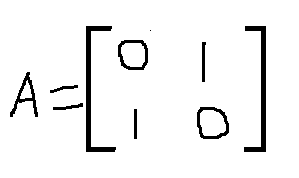如图A是一个置换矩阵，交换x的两个元素，什么样的x经过置换矩阵A作用后平行于x呢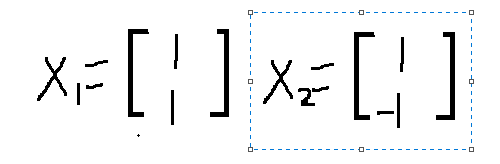λ1=1；λ2=-1；

提前说一个关于特征值的性质

nbyn矩阵有n个特征值，n>2时找出特征值并不容易，我们会得到高次方程，这里有个很好的性质。
特征值的和等于对角线元素的和，这个和称为
比如上面我知道了特征值1，可以立马求出另一个。
我们应该来再次来面对Ax=λx了
How to solve Ax=λx
用此技巧
（A-λI）x=0,I为单位矩阵
我们想，这个矩阵必须满足什么？
不考虑x=0，零向量是无用的特征向量，不考虑
（）内的矩阵必须时奇异的，不然无解
奇异矩阵的性质-行列式为0
|A-λI|=0
这是个关键方程，称为特征方程
可以解出n个λ
怎么样求解x，消元法即可

矩阵越特殊，特征值就越特殊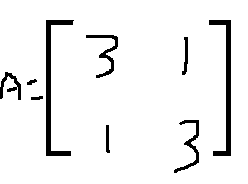比如上面的它的特征向量是垂直的，实际上必须是，待会再讲解为什么。

|A-λI|=（3-λ）2-1
=0
2-6λ+8
其中6为迹，8为行列式
λ1=4
λ2=2
带回去找x1和x2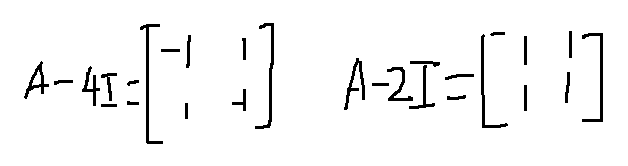即找他们零空间中的一个向量，显然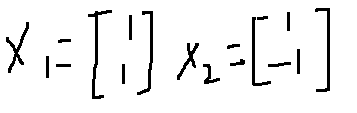这样我们得到了这个矩阵的特征值和特征向量，回到之前那个问题

A=[0 1;1 0]
特征向量为λ1=[1;1]
λ2= [1;-1]
这两个矩阵什么关系
一个比另一个多3I，这对特征值和特征向量什么影响呢？
特征值分别加了3，特征向量不变
why?
Ax=λx
(A+3I)x=λx+3x=(λ+3)x
下面是一个反例
Ax=λx
B有一个特征值α
A+B的特征值是什么？
Bx=αx
所以得到
（A+B）x=(α+λ)x
it’s not great!
我们没理由相信x也是B的特征向量，相加就无从谈起
如果B是单位矩阵的倍数，是没问题的，但多数情况B是一个普通的
下面再讲一个例子，
一个90°旋转矩阵Q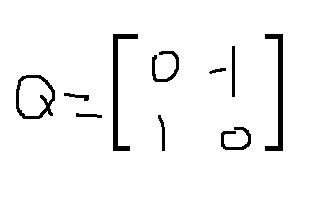我们对它的特征值和向量进行求解
根据迹我们知道
两个特征值之和=0；特征值之积=行列式；
λ1+λ2=0+0 ； λ1λ2=1；
回到这个矩阵，这个矩阵存在问题，什么向量旋转后和自己平行？
我们想象不出来旋转后的向量和原向量平行。我们再从计算行列式的方法重新来一遍
|Q-λI|=det[-λ -1;1 λ]=λ2+1=0
λ1=i;λ2=-i;
它们不是实数，即使矩阵里都是实数
特征值是一对共轭复数，这可能是完全实矩阵的特征值
事实上，如果矩阵是对称的或者接近对称的，那么特征值是实数
越不对称，越接近反对称，特征值越越是虚数，反对称矩阵（如此题）特征值为纯虚数，这是两种极端，介于对称和反对称之间的矩阵，部分对称部分反对称。
还有更糟糕的情况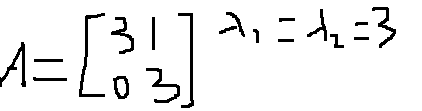为什么糟糕呢？让我们来看它的特征向量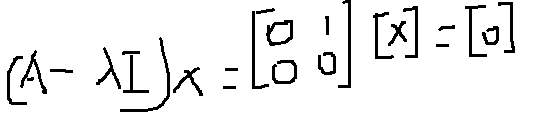x1=[1;0]但是无法找到另一个和x1线性无关的特征向量x2,只有一个方向的特征向量，
这是个2by’2的矩阵，只有一个特征向量

展开全文• 方阵A的特征值和特征向量为标量λ和满足条件的非零向量v关=λv在这个等式中, A是一个n×n矩阵, v是非零n×1向量, 而λ是标量(可以是实数也可以是复数)。该方程式具有其解的任何λ值都称为矩阵A的特征值。它也称为...
• QR法求解特征值特征向量

千次阅读 2021-04-05 20:58:48
当Ak基本收敛到为上三角矩阵时，迭代完成，此时主对角元素就是特征值。 特别地：当A是对称阵的时候，Ak是对角阵Λ，Q=Qk-1Qk-2…Q1就是其正交特征向量矩,有QTAQ=Ak=Λ，即A正交对角化与Ak。 如何理解？我们看下图...线性代数 机器学习 python
• 定义 对于给定的线性变换AAA,它的特征向量vvv经过这个线性变换之后，得到的新向量仍然与原来的 vvv保持在同一条直线上， 但其长度...λ 为标量， 即特征向量的长度在该线性变换下缩放的比例， 称 λ 为其特征值 。 ...特征值 特征向量
• 本文主要讲解利用Eigen库计算矩阵的特征值及特征向量并与Matlab计算结果进行比较。C++Eigen库代码#include #include #include using namespace Eigen;using namespace std;void Eig(){Matrix3d A;A << 1, 2, 3...
• 什么是特征值和特征向量： 在上一次线性代数学习之行列式 - cexo - 博客园学习了行列式相关的一些概念，其中也多次提到学好行列式是为了学习“特征值和特征向量”的基础，所以此次就正式进入这块内容的学习，也是...线性代数 矩阵
•python 特征值和特征向量
• 问题描述：MATLAB计算矩阵特征值和特征向量矩阵1：1 1/5 1/3 1/35 1 5 53 1/5 1 1/33 1/5 3 1矩阵2：1 3 1 5 1/7 71/3 1 3 1/3 1/9 51 1/3 1 1/3 1/5 71/5 3 3 1 1/5 57 9 5 5 1 91/7 1/5 1/7 1/5 1/9 1矩阵3：1 5 1...
• 特征值和特征向量计算器为4X4的实矩阵λ 是 [A] 矩阵的特征值 (标量)，如果有一个非零向量 (v) 这样满足以下关系:[A](v) = λ (v)每个向量 (v) 满足这个方程叫做 [A] 属于特征值 λ 的特征向量。作为一个例子，在一...
• -s V2[i,i+1] = s V2[i+1, i+1] = c ai2 = V2*ai2 ai2 = ai2*V1.T*V2.T print('迭代88次后得：') print(ai2) print('矩阵的特征值为{:.7},{:.7}，{:.7}'.format(ai2[0, 0],ai2[1, 1],ai2[2, 2]))特征值与特征向量
• 对比一下numpy与matlab求解的特征值与特征向量特征值 λ1=λ2=1 λ3=2特征值 λ1=-1 λ2=λ3=2如何求解特征向量1.这里列举第一个中λ=1时对应的特征向量。(A-λ*E)*x = 0得(A-E)*x = 0对A-E进行 行变换 得[[-2,1,0],...
• #求矩阵特征值以及特征向量 eig_value,eig_vector=np.linalg.eig(rela) #这里将特征值从小到大排列，但是sorted_indices表示的是所在下标的索引 sorted_indices=np.argsort(a) #这样我们就能求得最小特征值所对应的...算法
• 矩阵的特征值和特征向量特征值的理解特征值 、特征向量、方阵的关系几何意义物理意义特征分解特征值和特征向量的含义的应用补充一点 特征值的理解 其实刚上大学的时候上的线性代数课上，也只是简单讲解了特征值和...线性代数python 回归
• 设A是n阶矩阵，如果存在数λ和n维非零列向量x使关系式Ax=λx,则称数λ是方阵A的特征值，非零向量x成为A的对应于特征值λ的特征向量。 矩阵A乘以列向量x，相当于对x作线性变换，所得仍是一列向量。如果x是A的特征...线性代数
• A3:b =0.9389 0.9389 0.93890.3143 -0.1571 + 0.2722i-0.1571 - 0.2722i0.1403 -0.0701 - 0.1215i-0.0701 + 0.1215ic =3.0858 0 ...
• 《(最新整理)Matlab实现隐式QR方法求解矩阵特征值特征向量》由会员分享，可在线阅读，更多相关《(最新整理)Matlab实现隐式QR方法求解矩阵特征值特征向量(5页珍藏版)》请在人人文库网上搜索。1、完整)Matlab实现隐式...隐式求解matlab
•特征值 特征向量
• 下面验证一下 具体特征值的求解过程如下(写的比较乱)对称矩阵 特征值 特征向量
• 特征的数学意义] 我们先考察一种线性变化，例如x,y坐标系的椭圆方程可以写为x2/a2+y2/b2=1，那么坐标系关于原点做旋转以后，椭圆方程就要发生变换。我们可以把原坐标系的(x,y)乘以一个矩阵，得到一个新的(x’,y’)...矩阵
• 特征值为6.0248-0.0124 + 0.3863i-0.0124 - 0.3863i-0.00000.00000.0000对应第一个特征向量为：0.08260.57820.38490.24780.45130.4956对应第二个特征向量为：0.0275 + 0.0301i0.1923 + 0.2110i0.1148 - 0.5...
• 二、举个例子1、计算特征值与特征向量2、用特征向量表示任意向量三、理解其他结论1、对角化分解2、矩阵的特征值分别是原矩阵特征值的倒数3、特征值为0,意味着不可逆4、通过解Ax⃗=λx⃗A\vec{x}=\lambda \vec{x}Ax=...线性代数 矩阵
• [V,D] = EIG(X) produces a diagonal matrix D of eigenvalues and afull matrix V whose columns are the corresponding eigenvectors sothat X*V = V*D.例如：>> X=[1 5 7;6 4 2; 9 4 1]X =1 5 76 ...
• 目的求一个实对称矩阵的所有特征值和特征向量。前置知识对于一个实对称矩阵$A$，必存在对角阵$D$和正交阵$U$满足$$D=U^TAU$$$D$的对角线元素为$A$的特征值，$U$的列向量为$A$的特征向量。定义$n$阶旋转矩阵G(p,q,\...
• 一、特征值与特征向量 教程观摩：b站视频 1、定义： 从数学上看，如果向量v与变换A满足Av=λv，则称向量v是变换A的一个特征向量，λ是相应的特征值。这一等式被称作“特征值方程”。 意思：一个矩阵,左乘一个向量......